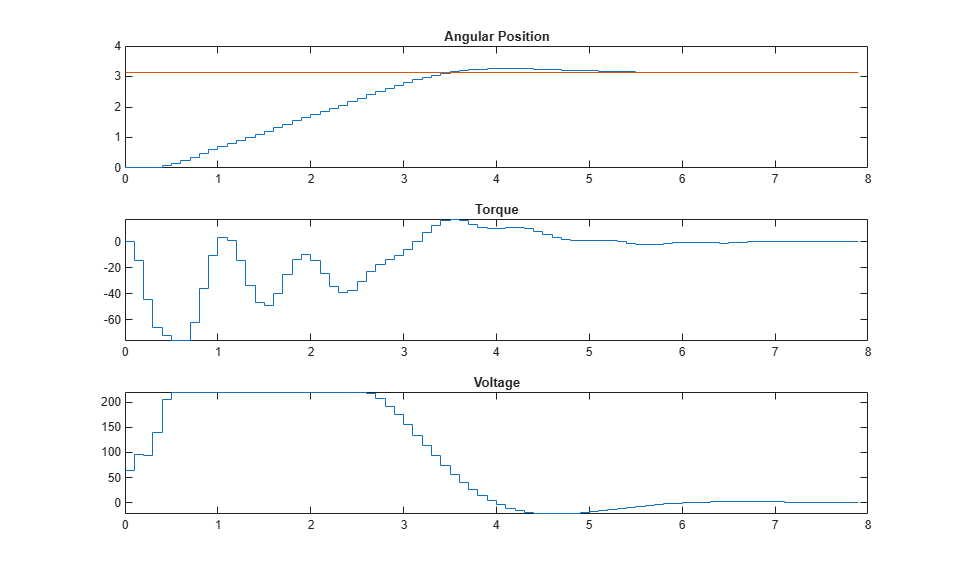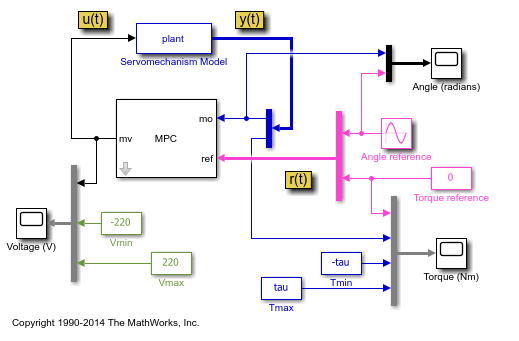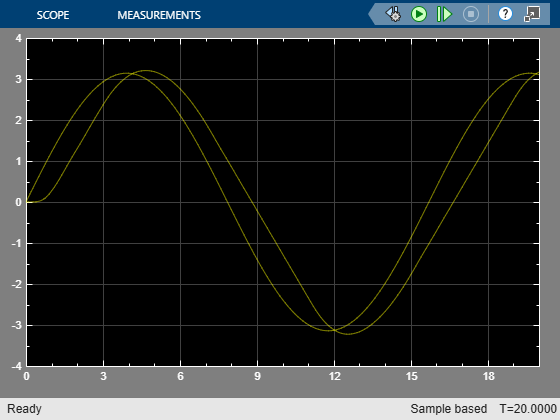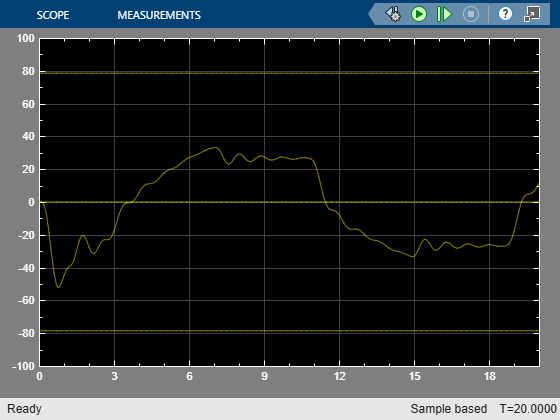Documentation

### This is machine translation

Mouseover text to see original. Click the button below to return to the English version of the page.

## DC Servomotor with Constraint on Unmeasured Output

This example shows how to design a model predictive controller for a DC servomechanism under voltage and shaft torque constraints.

Reference

 A. Bemporad and E. Mosca, ''Fulfilling hard constraints in uncertain linear systems by reference managing,'' Automatica, vol. 34, no. 4, pp. 451-461, 1998.

### Define DC-Servo Motor Model

The linear open-loop dynamic model is defined in "plant". Variable "tau" is the maximum admissible torque to be used as an output constraint.

```[plant, tau] = mpcmotormodel; ```

### Design MPC Controller

Specify input and output signal types for the MPC controller. The second output, torque, is unmeasurable.

```plant = setmpcsignals(plant,'MV',1,'MO',1,'UO',2); ```

MV Constraints

The manipulated variable is constrained between +/- 220 volts. Since the plant inputs and outputs are of different orders of magnitude, you also use scale factors to facilitate MPC tuning. Typical choices of scale factor are the upper/lower limit or the operating range.

```MV = struct('Min',-220,'Max',220,'ScaleFactor',440); ```

OV Constraints

Torque constraints are only imposed during the first three prediction steps.

```OV = struct('Min',{-Inf, [-tau;-tau;-tau;-Inf]},'Max',{Inf, [tau;tau;tau;Inf]},'ScaleFactor',{2*pi, 2*tau}); ```

Weights

The control task is to get zero tracking offset for the angular position. Since you only have one manipulated variable, the shaft torque is allowed to float within its constraint by setting its weight to zero.

```Weights = struct('MV',0,'MVRate',0.1,'OV',[0.1 0]); ```

Construct MPC controller

Create an MPC controller with plant model, sample time and horizons.

```Ts = 0.1; % Sampling time p = 10; % Prediction horizon m = 2; % Control horizon mpcobj = mpc(plant,Ts,p,m,Weights,MV,OV); ```

### Simulate Using SIM Command

Use `sim` command to simulate the closed-loop control of the linear plant model in MATLAB.

```disp('Now simulating nominal closed-loop behavior'); Tstop = 8; % seconds Tf = round(Tstop/Ts); % simulation iterations r = [pi*ones(Tf,1) zeros(Tf,1)];% reference signal [y1,t1,u1] = sim(mpcobj,Tf,r); ```
```Now simulating nominal closed-loop behavior -->Converting model to discrete time. Assuming no disturbance added to measured output channel #1. -->The "Model.Noise" property of the "mpc" object is empty. Assuming white noise on each measured output channel. ```

Plot results.

```subplot(311) stairs(t1,y1(:,1)); hold on stairs(t1,r(:,1)); hold off title('Angular Position') subplot(312) stairs(t1,y1(:,2)); title('Torque') subplot(313) stairs(t1,u1); title('Voltage') ```To run this example, Simulink® is required.

```if ~mpcchecktoolboxinstalled('simulink') disp('Simulink(R) is required to run this example.') return end ```

Simulate closed-loop control of the linear plant model in Simulink. Controller "mpcobj" is specified in the block dialog.

```mdl = 'mpc_motor'; open_system(mdl) sim(mdl) ```The closed-loop response is identical to the simulation result in MATLAB.

```bdclose(mdl) ```